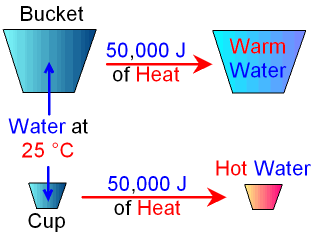gcsescience.com                                       3                                       gcsescience.com

Energy Transfer

What is the Difference between Heat and Temperature?

What is Heat?

Heat is a form of energy and is measured in joules.

Scientists use the word "thermal" to mean "heat".
Heat energy and thermal energy both mean the same thing.

What is Temperature?

Temperature is a measure of how hot something is
and is measured in °C (degree Celsius).
Heat is related to temperature but the two are not the same.

Imagine that you have a
bucket full of water and a cup full of water both at 25 °C.
If you add the same amount of heat energy
(for example 50,000J) to both you would find that
the temperature of the cup of water increases by
much more than the temperature of the bucket of water.Temperature is a measure of the kinetic energy of the particles
(the faster they are going, the hotter they are).
Temperature does not depend on the mass of the substance
(how many particles there are).

The amount of heat energy that a substance has
does depend on its mass.
If you double the mass, you must double the heat energy
to heat it to the same temperature.

The amount of heat energy that a substance has
also depends on its specific heat capacity.

What is Specific Heat Capacity?

The specific heat capacity is the amount of heat energy
needed to raise 1 kg of a substance by 1
°C.

Heat transferred = specific heat capacity x mass x change in temperature.

E = c x m x θ

Where E is the energy (heat) transferred in J

c is the specific heat capacity in J / kg °C

m is the mass in kg

θ (pronounced "theeter") is the change in temperature in °C .

This means that the same mass of different substances
will contain different amounts of heat energy
even though they are at the same temperature.Links         Energy Transfer         Revision Questionsgcsescience.com          Physics Quiz          Index          Heat Quiz          gcsescience.com